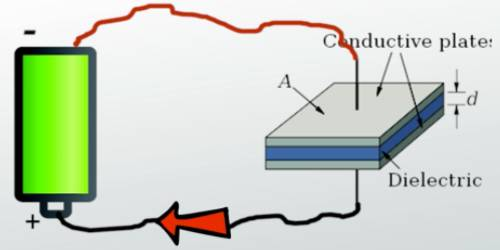# Factors on which Capacity of Conductor Depends

The capacitance of conductor is directly proportional to the area of plates and capacitance is inversely proportional to the distance between the plates.

Factors on which capacity of a conductor depends

According to the definition of capacitance, C = Q/V. So for a fixed amount of charges, the factors on which potential of a conductor change, the capacitance also will change for those factors. Followings are the causes of change:

(a) Area of the conductor: The more the area increases, the more will be the increase in capacitance. We know for a spherical conductor, C = 4πε0εrr. So as r increases, so area also increases, along with its capacitance C also will increase. It is generally true for all conductors.

(b) Medium surrounding the conductor: Capacitance of a conductor depends on the dielectric constant of the medium surrounding it. The capacitance of a conductor is more of the medium having higher dielectric constant and capacitance will be less for the medium having lower dielectric constant. If the capacitance of a conductor in air medium or in a vacuum is C0 and it is Ck for a medium of dielectric constant εr then from equation (Capacitance of a capacitor) it can be shown that,

εr = Ck/C0

= [Capacitance of a conductor in any medium / Capacitance of that conductor in the air or in vacuum]

(c) The separation between the plates: a Capacitance decrease due to the increase of separation between the plates of a parallel plate capacitor and reverse is true when separation decreases.

(d) Presence of the conductor with any other conductor or grounded conductor: If there exists any other changeless or grounded conductor with the charged conductor, then due to electrical induction, the capacitance of the experimental conductor will increase. But if a similarly charged body is present near the charged conductor, the capacitance of the experimental conductor will decrease and if the oppositely charged body is present then capacitance will increase.

There are several factors on which resistance depends which are the following: The resistance of a conductor first depends on the material from which it is made. The resistance is nothing but opposition in the path of free electrons of the conductor.

• Resistivity: Resistance depends upon resistivity which is proportionality constant and depends on the nature of substance and temperature.
• Temperature: The temperature is a vital factor in determining the resistance of the conductor. As the temperature increases, the value of resistivity increases so resistance increases. Thus in the case when the temperature increases, resistance decreases.
• Length: Resistance is directly proportional to the length. So as the length increases the value of resistance increases.
• Specific Conductivity: This property refers to how much the substance will conduct. This property is fixed for a fixed material.
• Cross Section Area: Resistance is inversely proportional to the cross-section area. More the area of cross-section, the resistance is less. So as the cross-section area increases the value of resistance decreases. Similarly, the length of directly proportional to the length of the conductor. More the length, the more the resistance.